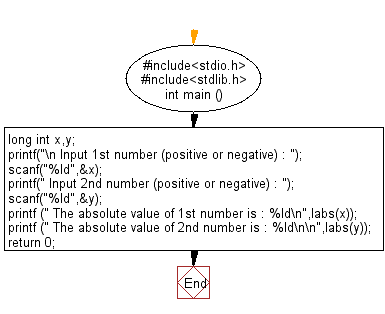﻿ C Program: Return the absolute value of a long integer - w3resource# C Exercises: Return the absolute value of a long integer

## C Variable Type : Exercise-8 with Solution

Write a C program to return the absolute value of a long integer.

Sample Solution:

C Code:

``````#include<stdio.h>
#include<stdlib.h>

int main ()
{
long int x,y;
printf("\n Input 1st number (positive or negative) : ");
scanf("%ld",&x);
printf(" Input 2nd number (positive or negative) : ");
scanf("%ld",&y);
printf (" The absolute value of 1st number is : %ld\n",labs(x));
printf (" The absolute value of 2nd number is : %ld\n\n",labs(y));

return 0;
}
```
```

Sample Output:

```Input 1st number (positive or negative) : 25
Input 2nd number (positive or negative) : -125
The absolute value of 1st number is : 25
The absolute value of 2nd number is : 125
```

Flowchart:C Programming Code Editor:

Improve this sample solution and post your code through Disqus

What is the difficulty level of this exercise?

Test your Programming skills with w3resource's quiz.

﻿

## C Programming: Tips of the Day

How I can print to stderr in C?

The syntax is almost the same as printf. With printf you give the string format and its contents i.e.:

`printf("my %s has %d chars\n", "string format", 30);`

With fprintf it is the same, except now you are also specifying the place to print to:

```File *myFile;
...
fprintf( myFile, "my %s has %d chars\n", "string format", 30);```

`fprintf( stderr, "my %s has %d chars\n", "string format", 30);`